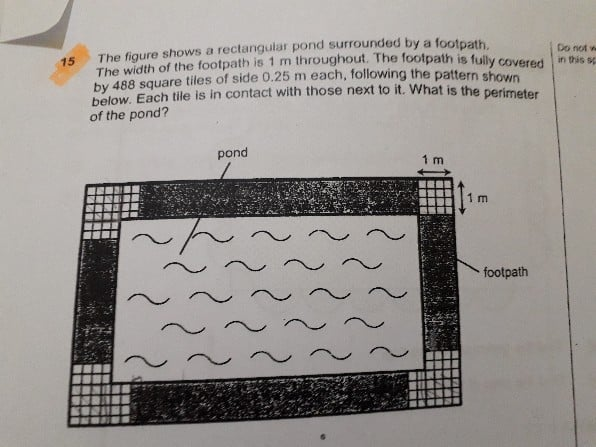# QuestionHow to solve

Due to the square at the corners, we do not have 4 equal sets of squares. In order to have 4 equal sets of squares, we need to first remove the squares from the corners

4 x 4 = 16 (Number of squares in 1 corner)

4 x 16 = 64 (Number of squares in 4 corners)

488 – 64 = 424 (Total squares without the squares at 4 corners)

424 is also the remaining number of squares along 2 lengths and 2 breadths. Since there are 4 layers of squares, we need to next divide by 4 to find the number of squares in 1 layer

424 ÷ 4 = 106

Since there are 106 squares along 2 lengths and 2 breadths, we can also conclude that there are 106 sets of 0.25 m along 2 lengths and 2 breadths

106 x 0.25 m = 26.5 m (Perimeter of the pond)

0 Replies 0 Likes